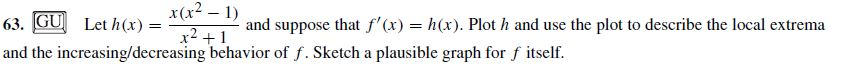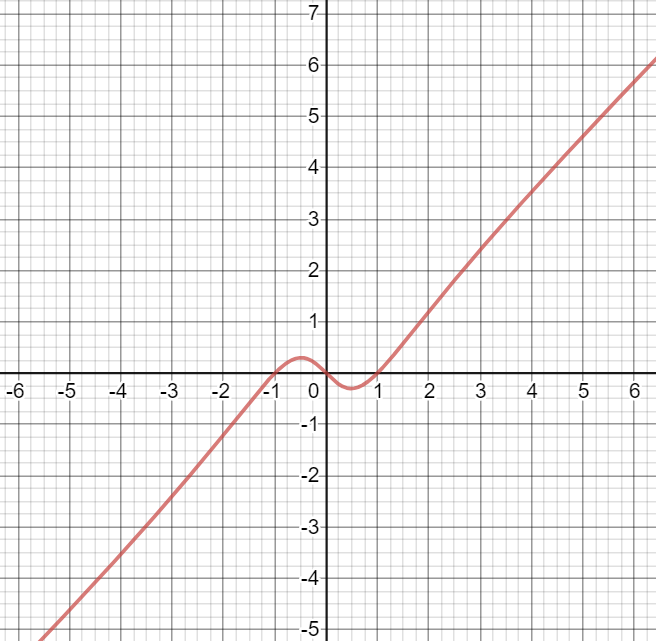# x(x2 – 1)x2 +163. GU Let h(x)and the increasing/decreasing behavior of f. Sketch a plausible graph for f itself.and suppose that f'(x) = h(x). Plot h and use the plot to describe thelocal extrema

Question
4 viewshelp_outlineImage Transcriptionclosex(x2 – 1) x2 +1 63. GU Let h(x) and the increasing/decreasing behavior of f. Sketch a plausible graph for f itself. and suppose that f'(x) = h(x). Plot h and use the plot to describe the local extrema fullscreen
check_circle

Step 1

We use a graphing calculator to graph h(x)Step 2

The x-intercepts are at x=-1, x=0 and x=1

Those are the critical points of f(x).

When h(x) is negative then f(x) will be decreasing and when h(x) is positive then f(x) will be increasing.

h(x) is negative in (-infinity,-1) and (0,1)

So, in (-infinity,-1) and (0,1) f(x) is decr...

### Want to see the full answer?

See Solution

#### Want to see this answer and more?

Solutions are written by subject experts who are available 24/7. Questions are typically answered within 1 hour.*

See Solution
*Response times may vary by subject and question.
Tagged in